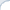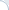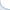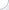This post has a couple of updates. Make sure to check my notes on plotting a recall-precision curve for k-NN search, especially, for the case when distances are computed exactly.

In certain situations, an average precision is equal to recall. Proving that this is the case and finding ways to deal with this issue can be a good problem for an IR course homework and might help students to grok the notion of the average precision. This problem was inspired by the real research paper where authors used the mean average precision without realizing it was equal to recall.

Imagine that a nearest-neighbor search should retrieve 10 images most similar to a query image. The search is typically not ideal and some found images would be quite different from the query image. If we make humans judge retrieved results, it can be possible to evaluate search effectiveness via the (mean) average precision. If human judgments are not available, one may decide to use a proxy metric: An image is relevant if it is a true nearest neighbor, i.e., it is one of the 10 most closest images with respect to the values of the distance function. An exact search method always returns the true 10 nearest neighbors, but an approximate method may fail to do so. A degree of "sloppiness" can be estimated using the average precision, right?

I think that this wrong and here is why. In this formulation, a retrieved image is relevant when a distance from this image to the query object does not exceed the distance from the query image to the 10-th true nearest neighbor. Furthermore, all returned objects are normally sorted in the order of increasing distance from the query. Consequently, there will be $R\le10$ objects with distances not exceeding the distance to the 10-th nearest neighbor. And these images will clearly be considered "relevant". The following $10-R$ images will have distances larger than the distance to the 10-th nearest image and, thus, they will not be considered relevant.

It is not hard to see that in this case the average precision is equal to $R/10$ (by the definition of the average precision, P@N is one if $N \le R$ and is zero otherwise). However, the recall (the fraction of true nearest neighbors returned) is also $R/10$.
A good homework problem can ask the following:

1) To prove the equality of the average precision and the recall (the precision is perfect for each relevant element retrieved);
2) To describe ways of dealing with this issue, i.e., can we still use the average precision (after some fixes)? If not, what else can be used?

The second question is clearly open-ended, because (in addition to introducing real human judgments) multiple solutions are possible, which include but are not limited to using a rank approximation error.

Update/Addition #1: The situation is different if distances are computed only approximately, i..e, if the order of returned neighbors is not necessarily correct. For example, if we retrieve 5 nearest neighbors out of 10 and rank them as 1st, 2d, 4th, ..., 10th. In this case, computing precision makes a lot of sense.

Update/Addition #2: It can still make sense to plot a recall-precision curve. Assuming that the precision axis is $y$, for a single query, we get the perfect precision as recall increases from zero to $R/10$. Then, the precision would decrease linearly to $R/10$ while recall would stay constant at $R/10$. Clearly, the value of $R$ varies across queries. Thus, when we average over queries, we may still get some meaningful picture. For example, if there is a low variance in recall, the plot would be similar to a single-query recall-precision plot: a nearly horizontal line followed by a sharp vertical drop. If the variance is high, there will be a smoother downward curve. Thus, on second thought, in the case of the intrinsic evaluation, this plot can still be interesting despite the average precision is equal to the $k$-NN recall.